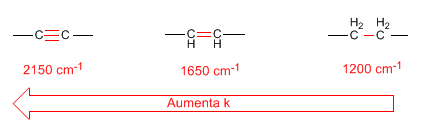As we commented in the previous point, the absorption frequency depends on the force constant and the mass of the vibrating atoms.

• Force constant. Triple bonds are stronger than double bonds, and double bonds are stronger than single bonds. Therefore, the force constant of triple bonds is greater than that of double bonds, with the force constant of single bonds being the smallest.• Hybridization of CH bonds also produces changes in the force constant according to the following list:• reduced mass. The decrease in the reduced mass produces a displacement of the absorption bands towards higher frequencies:• Tension vibrations come out at a higher number of waves than bending vibrations. (CH Tension: 3000$cm^{-1}$; CH Flexure: 1300 - 1400$cm^{-1}$)